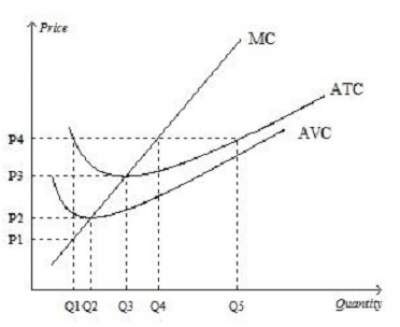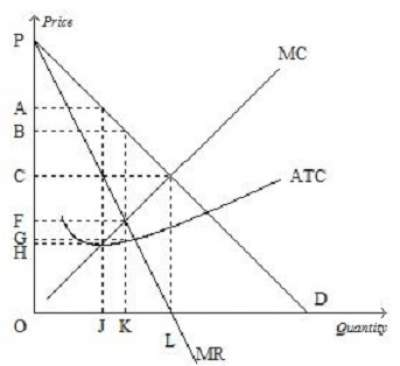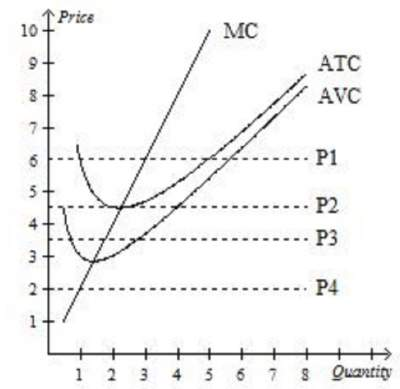# Overall Practice Test-1 on Economics

Question 1: Economics deals primarily with the concept of ..........
1. Money.
2. Scarcity.
3. Power.
4. Banking.
5. none of the above
Question 2: Which of the following statements is correct? .....
1. A competitive firm is a price maker, whereas a monopolist is a price taker.
2. Both a competitive firm and a monopolist are price makers.
3. A competitive firm is a price taker, whereas a monopolist is a price maker.
4. Both a competitive firm and a monopolist are price takers.
5. 1 and 2
Question 3: In a market economy, supply and demand determine.............
1. both the quantity of each good produced and the price at which it is sold
2. the price at which each good is sold, but not the quantity of each good produced.
3. neither the quantity of each good produced nor the price at which it is sold.
4. the quantity of each good produced, but not the price at which it is sold.
5. 1 and 4
Question 4: Which of the following is not a characteristic of a monopoly? ......
2. barriers to entry.
3. one seller.
4. a product without close substitutes.
5. 2 and 3
Question 5: When quantity demanded decreases at every possible price, we know that the demand curve has ......
1. shifted to the right.
2. shifted to the left.
3. not shifted; rather, we have moved along the demand curve to a new point on the same curve.
4. none of the above.
Question 6: The opportunity cost of going to college is .........
1. the value of the best opportunity a student gives up to attend college.
2. clothing, books, meals, transportation, tuition, lodging, and any other expenses.
3. zero for students who are fortunate enough to have all of their college expenses paid by someone else.
4. zero, since a college education will allow a student to earn a larger income after graduation.
Question 7: Which of the following statements is correct?
1. Sellers determine both demand and supply.
2. Buyers determine both demand and supply.
3. Buyers determine demand and sellers determine supply.
4. Buyers determine supply and sellers determine demand.
Question 8: Explicit costs
1. include income that is forgone by the firm's owners.
2. require an outlay of money by the firm.
3. include all of the firm's opportunity costs.
4. all of the above.
Question 9: Amy decides to open her own business and earns \$75,000 in accounting profit the first year.
When deciding to open her own business, she turned down two separate job offers with annual salaries of \$40,000, \$50,000, and \$60,000.
What is Amy's economic profit from running her own business?
1. \$35,000
2. \$20,000
3. −\$15,000
4. \$15,000
Assume that the table below shows the expenses of a local IT startup ABC.
Q TC FC VC MC AFC AVC ATC
0 \$150 \$150 \$0 .... .... .... ....
1 \$250 A B C D E F
2 G H I \$220 J K L
3 M N O P Q \$220 R

Question 10: Find A.
1. \$150
2. \$100
3. \$75
4. \$45
Question 11: Using the same table of the company ABC, find G.
1. \$255
2. \$265
3. \$470
4. \$275
Question 12: Based on the graph below when price rises from P3 to P4, the firm finds that........1. fixed costs decrease as output increases from Q3 to Q4.
2. it can earn a positive profit by increasing production to Q4.
3. profit is still maximized at a production level of Q3
4. average revenue exceeds marginal revenue at a production level of Q4
Question 13: Using the same figure in question 12, firms would be encouraged to enter this market for all prices that exceed .....
1. P2
2. P3
3. P4
4. P5
5. none of the above
Question 14: The fundamental source of monopoly power is ............
1. profit.
2. barriers to entry.
3. decreasing average total cost.
4. a product without close substitutes.
Question 15: See the graph below and find the mathematical formula to calculate the monopolist's profit.1. (B − C) × J
2. (A − G) × L
3. (B − C) × K
4. (B − G) × K
5. none of the above
Question 16: A profit-maximizing monopoly's will produce an output equal to .......1. Q3
2. Q1
3. Q2
4. Q4
Question 17: Which of the following pairs illustrates the two extreme examples of market structures?
1. competition and monopoly
2. competition and oligopoly
3. monopoly and monopolistic competition
4. oligopoly and monopolistic competition